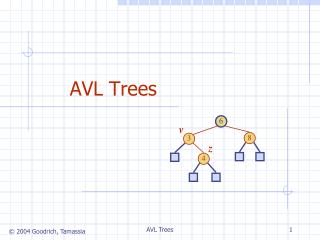DownloadDownload PresentationAVL Trees

# AVL Trees

Télécharger la présentation## AVL Trees

- - - - - - - - - - - - - - - - - - - - - - - - - - - E N D - - - - - - - - - - - - - - - - - - - - - - - - - - -
##### Presentation Transcript

1. 6 v 8 3 z 4 AVL Trees AVL Trees

2. AVL Tree Definition • AVL trees are balanced. • An AVL Tree is a binary search tree such that for every internal node v of T, the heights of the children of v can differ by at most 1. An example of an AVL tree where the heights are shown next to the nodes: AVL Trees

3. n(2) 3 n(1) 4 Height of an AVL Tree • Fact: The height of an AVL tree storing n keys is O(log n). • Proof: Let us bound n(h): the minimum number of internal nodes of an AVL tree of height h. • We easily see that n(1) = 1 and n(2) = 2 • For n > 2, an AVL tree of height h contains the root node, one AVL subtree of height n-1 and another of height n-2. • That is, n(h) = 1 + n(h-1) + n(h-2) • Knowing n(h-1) > n(h-2), we get n(h) > 2n(h-2). So n(h) > 2n(h-2), n(h) > 4n(h-4), n(h) > 8n(n-6), … (by induction), n(h) > 2in(h-2i) • Solving the base case we get: n(h) > 2 h/2-1 • Taking logarithms: h < 2log n(h) +2 • Thus the height of an AVL tree is O(log n) AVL Trees

4. 44 17 78 44 32 50 88 17 78 48 62 32 50 88 54 48 62 Insertion in an AVL Tree • Insertion is as in a binary search tree • Always done by expanding an external node. • Example: c=z a=y b=x w before insertion after insertion AVL Trees

5. a=z a=z T0 c=y T0 b=y b=x T3 c=x T1 b=y b=x T1 T2 T2 T3 a=z c=x a=z c=y T2 T3 T1 T3 T0 T0 T2 T1 Trinode Restructuring • let (a,b,c) be an inorder listing of x, y, z • perform the rotations needed to make b the topmost node of the three (other two cases are symmetrical) case 2: double rotation (a right rotation about c, then a left rotation about a) case 1: single rotation (a left rotation about a) AVL Trees

6. T T 1 1 Insertion Example, continued unbalanced... 4 44 x 3 2 17 62 z y 2 1 2 78 32 50 1 1 1 ...balanced 54 88 48 T 2 T T AVL Trees 0 3

7. Restructuring (as Single Rotations) • Single Rotations: AVL Trees

8. Restructuring (as Double Rotations) • double rotations: AVL Trees

9. 44 17 62 32 50 78 88 48 54 Removal in an AVL Tree • Removal begins as in a binary search tree, which means the node removed will become an empty external node. Its parent, w, may cause an imbalance. • Example: 44 17 62 50 78 88 48 54 before deletion of 32 after deletion AVL Trees

10. Rebalancing after a Removal • Let z be the first unbalanced node encountered while travelling up the tree from w. Also, let y be the child of z with the larger height, and let x be the child of y with the larger height. • We perform restructure(x) to restore balance at z. • As this restructuring may upset the balance of another node higher in the tree, we must continue checking for balance until the root of T is reached 62 44 a=z 44 78 17 62 w b=y 17 50 88 50 78 c=x 48 54 88 48 54 AVL Trees

11. Running Times for AVL Trees • a single restructure is O(1) • using a linked-structure binary tree • find is O(log n) • height of tree is O(log n), no restructures needed • insert is O(log n) • initial find is O(log n) • Restructuring up the tree, maintaining heights is O(log n) • remove is O(log n) • initial find is O(log n) • Restructuring up the tree, maintaining heights is O(log n) AVL Trees